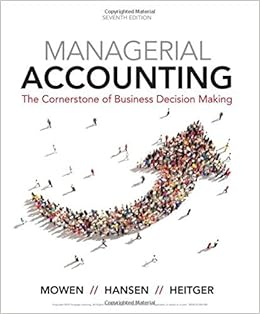Answer the following questions using the information

• Test Prep
• 68
• 96% (674) 648 out of 674 people found this document helpful

This preview shows page 42 - 45 out of 68 pages.

We have textbook solutions for you!
The document you are viewing contains questions related to this textbook.The document you are viewing contains questions related to this textbook.
Chapter 11 / Exercise 11-34
Managerial Accounting: The Cornerstone of Business Decision-Making
Hansen/MowenExpert Verified
Answer the following questions using the information below: These questions refer to flexible-budget variance formulas with the following descriptions for the variables: A = Actual; B = Budgeted; P = Price; Q = Quantity. 23) Which variance is calculated using the formula (AQ - BQ) BP is the ________. A) efficiency variance B) price variance C) total flexible-budget variance D) spending variance Answer: A Diff: 2
Objective: 5 AACSB: Analytical thinking 24) Which variance is calculated by using the formula: (AP - BP) AQ is the ________. B Diff: 2
Objective: 5 AACSB: Analytical thinking
We have textbook solutions for you!
The document you are viewing contains questions related to this textbook.The document you are viewing contains questions related to this textbook.
Chapter 11 / Exercise 11-34
Managerial Accounting: The Cornerstone of Business Decision-Making
Hansen/MowenExpert Verified
25) The flexible-budget variance for materials is \$2,000 (U). The sales-volume variance is \$18,000 (U). The price variance for material is \$38,000 (F). The efficiency variance for direct manufacturing labor is \$12,000 (F). Calculate the efficiency variance for materials. D Diff: 3
Objective: 5 AACSB: Application of knowledge 26) Which of the following is the correct formula for the materials price variance? C Diff: 2
Objective: 4 AACSB: Analytical thinking 27) A company purchases \$650,000 of materials on credit. The standard cost for the materials is \$675,000. Which of the following would be the correct journal entries to record the purchase under a standard costing system? A) Direct Materials Control \$675,000 Direct Materials Price Variance \$25,000 Accounts Payable Control \$650,000 B) WIP Control \$675,000 Direct Materials Price Variance \$25,000 Accounts Payable Control \$650,000 C) Accounts Payable Control \$650,000 Direct Materials Price Variance \$25,000 Direct Materials Control \$675,000 D) Accounts Payable Control \$650,000 Direct Materials Price Variance \$25,000 WIP Control \$675,000 Answer: A Diff: 1
Objective: 5 AACSB: Analytical thinking
28) These questions refer to flexible-budget variance formulas with the following descriptions for the variables: A = Actual; B = Budgeted; P = Price; Q = Quantity. The best label for the formula [(AP)(AQ) - (BP)(BQ)] is the ________. C Diff: 2
Objective: 5 AACSB: Analytical thinking
•••